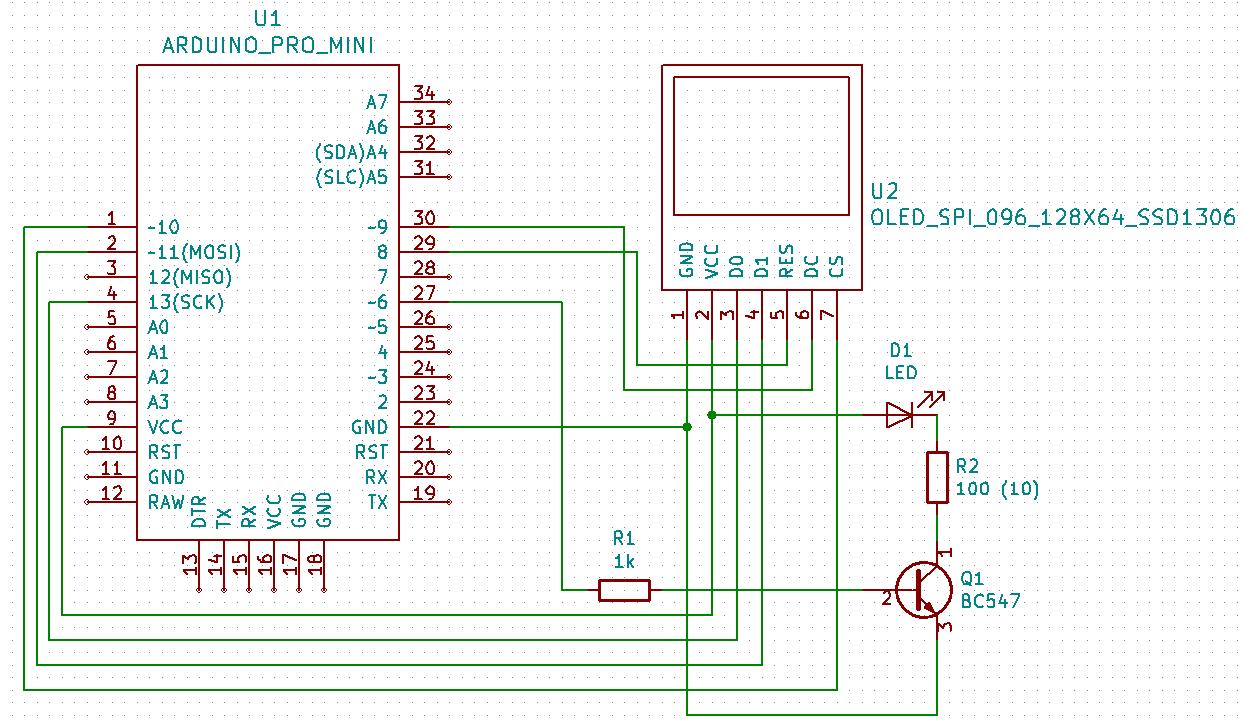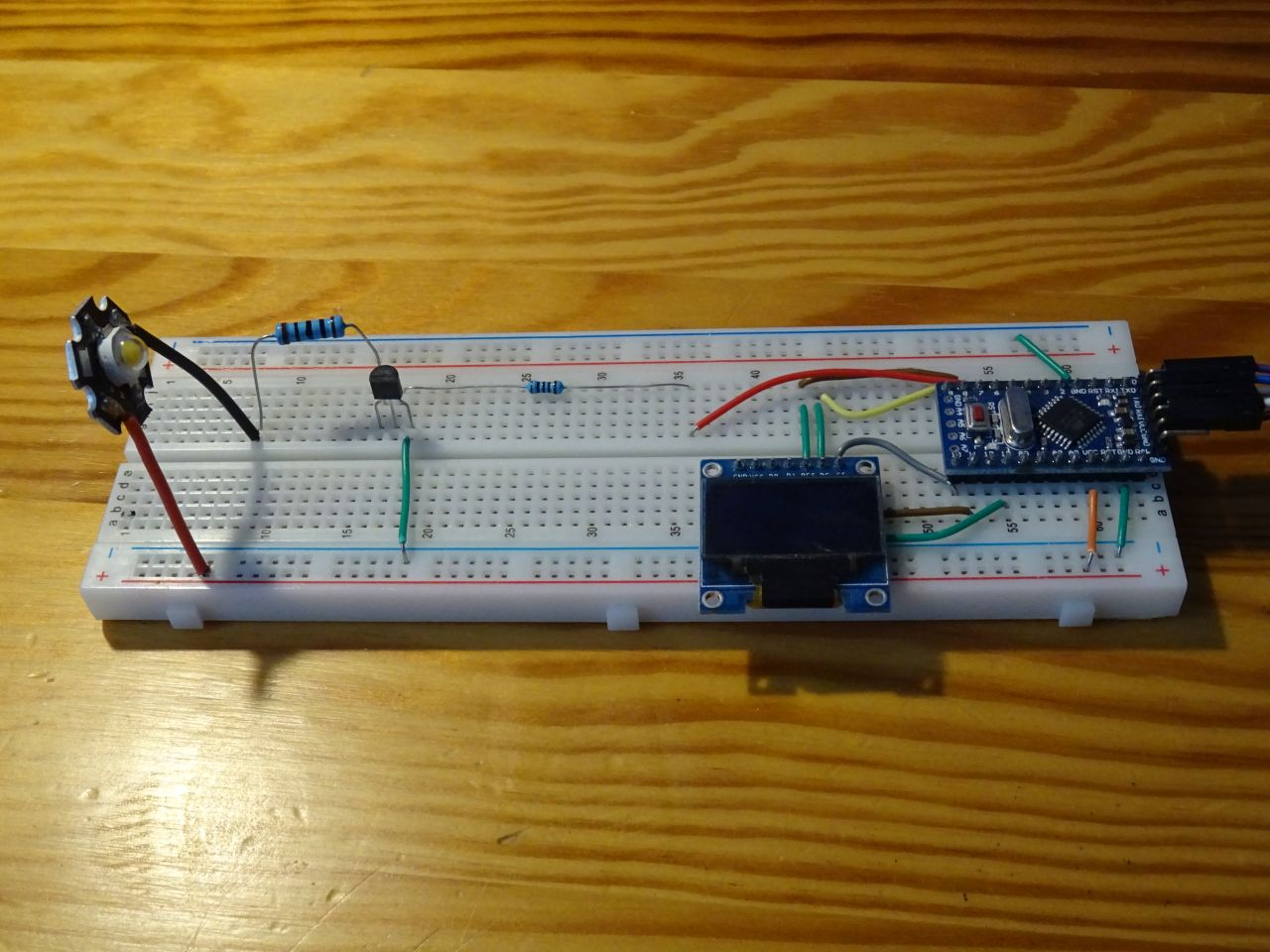# Arduino and bipolar transistor as a switch

Zápisník experimentátora

In this article, we will explain how we can strengthen the Arduino output pin using a transistor. The purpose of the article is not to explain the theory of transistor operation. We will limit ourselves to the minimum necessary. As an example, we use a high-light LED, which can flow 350 mA. This is far beyond the possibilities of Arduino. We will be able to control it with a transistor.

## Components

We need these parts.

• Resistor 100R, 10R, 1k {linkR}
• Wires## Bipolar transistor

The bipolar transistor works by connecting the Arduino output pin to the transistor base. You must limit the overcurrent by using a resistor. Since the BC547B resistor used has an amplification factor of approximately 200, a 1k resistor can be used. If you would like to calculate a value, you can do so using a simple formula. The open transistor voltage between the base and emitter is approximately 0.7V. To make sure the transistor opens, we'll use three times what we need to be sure.

`(5V - 0,7V) / 1000 * 200 / 3 = 0.28A`

Small wiring note. We should connect one more 100k R3 resistor to the transistor base. Nothing bad happens when you engage on a breadboard. However, Arduino has its outputs when connected to a power supply in high impedance. This means that they are not connected to either VCC or GND. They're like in the air. And because it is enough to bring a small current to the base to turn the transistor on, it may happen that the diode lights up for a while before the Arduino sketch starts.## Program

I also used OLED display. In this particular case of LED flashing is not necessary, but I am already preparing the material for the following article. In this case, the display only shows the current status based on the transistor. In the program I use the TimerOne library to flash the LEDs at half-second intervals.

``````#include <U8g2lib.h>
#include <TimerOne.h>

U8G2_SSD1306_128X64_NONAME_1_4W_HW_SPI u8g2(U8G2_R0, /* cs=*/ 10, /* dc=*/ 9, /* reset=*/ 8);

#define ledPin 6
int ledState = LOW;

void setup() {
u8g2.begin();
u8g2.setFont(u8g2_font_ncenB10_tr);

pinMode(ledPin, OUTPUT);
Timer1.initialize(500000); // 500 ms
}

void loop() {
for (int i = 12; i < 64 - 24; i++) {
drawFrame(i);
delay(10);
}
for (int i = 63 - 24; i >= 12; i--) {
drawFrame(i);
delay(10);
}
}

void drawFrame(int y) {
u8g2.firstPage();
do {

u8g2.drawStr(0, y, "Arduino Slovakia");
u8g2.drawStr(8, y + 24, ledState ? "ON" : "OFF");
for (int j = 0; j <= 128; j += 2)
u8g2.drawLine(j, 0, j, (j % 10 == 0 ? 2 : 1));
for (int j = 0; j <= 64; j += 2)
u8g2.drawLine(0, j, (j % 10 == 0 ? 2 : 1), j);

} while ( u8g2.nextPage() );
}